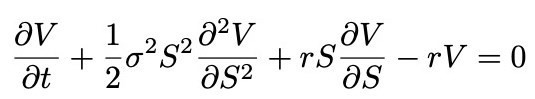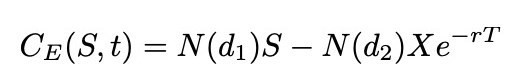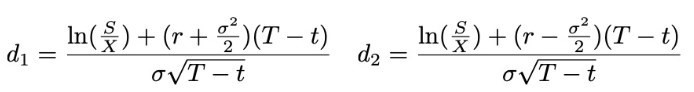# Definition of the Black-Scholes model – What is Black-Scholes?

The Black-Scholes model, sometimes referred to as the Black-Scholes-Merton model, is a mathematical model using six variables to calculate the theoretical value of a European-style option contract. Developed in 1973, the Black-Scholes model of option pricing remains relevant today for hedging option portfolios to reduce the risk profile by buying and selling the underlying asset. It is also known as continuously revised delta coverage.

## The Black-Scholes model explained

According to the Black-Scholes model, asset prices have a log-normal distribution, experience continuous drift and volatility, and follow random walk theory, suggesting that technical analysts cannot predict future price movements. This is still a widely discussed topic, but the Black-Scholes model starts with a parabolic partial differential equation called the Black-Scholes equation, from which users can derive the Black-Scholes formula.

## The Black-Scholes model requires the following parameters:

• The current share price
• Expected dividends
• The exercise price of the option
• Expected interest rates
• Expiration date
• Expected volatility

The simple explanation of the Black-Scholes model is that it provides a theoretical estimate of European-style option prices. It aims to illustrate that an option contract has a single price unrelated to the risk of the asset and its expected return. The Black-Scholes model replaces the expected return of the underlying asset with a risk-neutral rate. Some describe the Black-Scholes model as a second-order partial differential equation.

## A historical overview of the Black-Scholes model

American economist Fischer Black and Canadian-American financial economist Myron Scholes developed the Black-Scholes model in 1973. They introduced it in their 1973 article “The Pricing of Options and Corporate Liabilities”, published in the Journal of Political Economy.

American economist Robert Merton contributed to the writing of the article and published his article “Theory of Rational Option Pricing” in The Bell Journal of Economics and Management Science. He coined the term Black-Scholes Theory of Options Pricing and expanded the mathematical understanding and applications of the model, which remains in use today, primarily for hedging and risk mitigation strategies, with variations and relaxations rules.

Scholes and Merton received the Nobel Prize in Economics in 1997, as Black died in 1995, and the Nobel Foundation does not award prizes posthumously. He acknowledged Black’s contributions to the development of the Black-Scholes model.

## Applications of the Black-Scholes model

The Black-Scholes model is used in one of its many model modifications and extensions for hedging and risk mitigation by investment banks, hedge funds, professional traders, broker-dealers and retail traders advances.

### Some of the applications of the Black-Scholes model include:

• Put and call options
• Futures contracts
• OTC derivatives
• Assets with continuously yielding dividends (modified model), e.g. index options
• Asset with discrete proportional dividends (modified model), e.g. single stock options
• American options (modified model)
• Perpetual put options
• Binary options and their relationship to vanilla options
• Cash or nothing call/sell options
• Call/put options with or without assets
• Currency options

## The Black–Scholes formula

Looking at any Black-Scholes model example is daunting because the Black-Scholes model formula is extremely complex and intimidating. Most will struggle to figure it out, but there are plenty of calculators and trading platform tools out there that handle the calculations and give traders the results.

The formula for the Black-Scholes parabolic partial differential equation is:Here is the Black-Scholes formula for a call option (C) on a non-dividend paying asset (S):The formulas for the inputs d₁ and d₂ are:Or:

• S = Price of the underlying asset
• T = expiration date
• t = Current date
• X = Strike price
• r = risk-free interest rate
• σ = Volatility (standard deviation of the underlying asset)
• NOT

## = Cumulative distribution function for a normal (Gaussian) distribution

Explanation of the assumptions of the Black-Scholes model

## Before using the Black-Scholes model, traders and risk managers should understand the assumptions of the Black-Scholes model, which correlate with the limitations of the Black-Scholes model.

• The basic assumptions of the Black-Scholes model are as follows:
• Lognormal distribution (following Brownian motion)
• No dividends (many model modifications and extensions exist to account for dividends)
• Random walk theory (a highly contested theory suggesting that technical analysis cannot predict future price movements)
• Frictionless market (an unrealistic assumption that there are no transaction costs)
• Risk-free interest rate (a false assumption that interest rates are a constant)
• Normal distribution (a false assumption that asset volatility is constant)

### No Arbitrage (a false assumption that arbitrage trading does not exist)

The limits of the Black-Scholes model

### The most notable limitations of the Black-Scholes model are its false or unrealistic assumptions about a frictionless market, the risk-free interest rate, the normal distribution, and the lack of arbitrage mentioned above. Assuming variables are constant and ignoring actual market conditions will lead to inaccurate results deviating from live price action. Dividend-adjusted models exist. Otherwise, the Black-Scholes model would provide unreliable option pricing.

Conclusion of the Black-Scholes model

## The original Black-Scholes model consists of four false or unrealistic assumptions, one highly contested and one debatable. Consequently, many applications of the Black-Scholes model used today include model modifications and extensions of the basic formula, which some consider to be the most efficient option pricing model available.

FAQs

Do traders use the Black-Scholes model?

Traders and risk managers use the Black-Scholes model for hedging and risk mitigation despite several faulty assumptions in the original formula.

How accurate is the Black-Scholes model?

Accuracy depends on modifications made to the original formula to correct false or unrealistic assumptions. Many consider a modified Black-Scholes model to be the most effective option pricing tool.

Categories Uncategorized